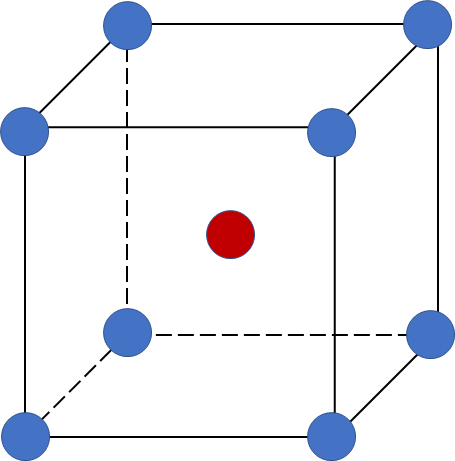# Problem: How many atoms are in the body-centered cubic unit cell? What’s its coordination number? What’s its packing efficiency?

###### FREE Expert Solution

A body-centered cubic (BCC) unit cell is composed of a cube with one atom at each of its corners and one atom at the center of the cubeWe’re going to do the following steps:

Step 1: Determine how many atoms are in BCC
Step 2: Determine the coordination number
Step 3: Calculate the packing efficiency

85% (137 ratings)###### Problem Details

How many atoms are in the body-centered cubic unit cell? What’s its coordination number? What’s its packing efficiency?

Frequently Asked Questions

What scientific concept do you need to know in order to solve this problem?

Our tutors have indicated that to solve this problem you will need to apply the Unit Cell concept. You can view video lessons to learn Unit Cell. Or if you need more Unit Cell practice, you can also practice Unit Cell practice problems.